# Recursion Recursion Idea Some problems can be broken

• Slides: 11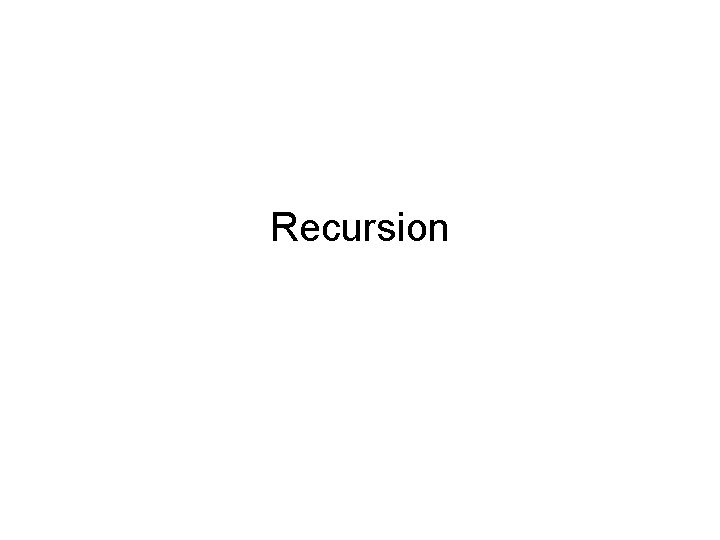Recursion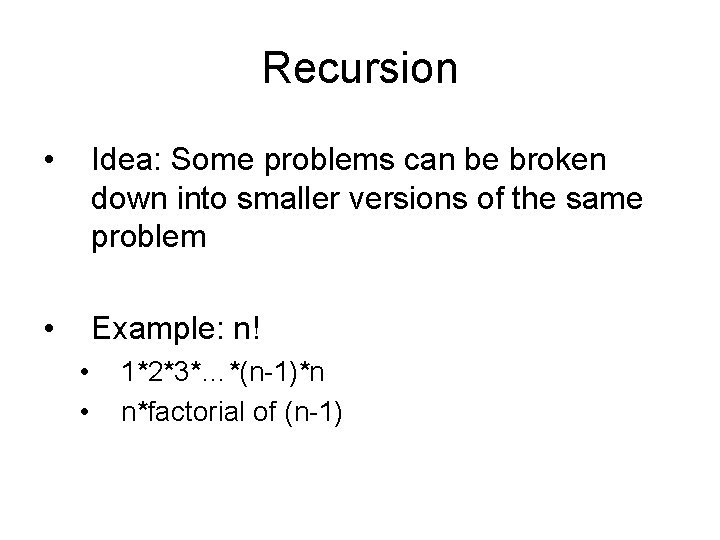Recursion • Idea: Some problems can be broken down into smaller versions of the same problem • Example: n! • • 1*2*3*…*(n-1)*n n*factorial of (n-1)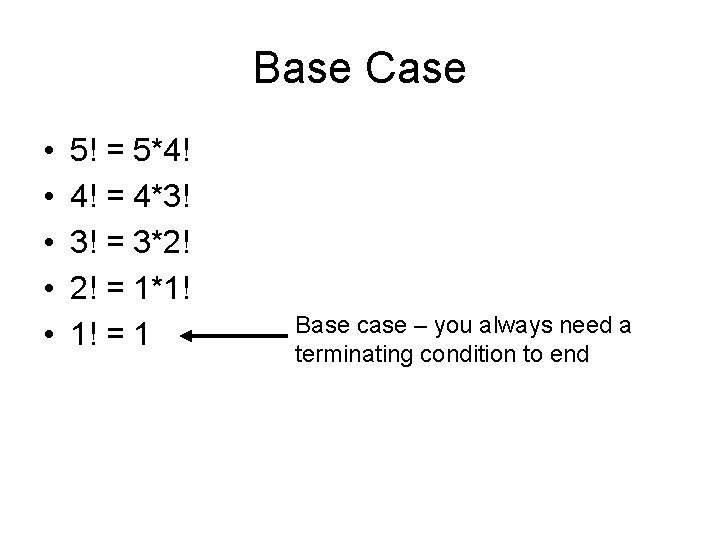Base Case • • • 5! = 5*4! 4! = 4*3! 3! = 3*2! 2! = 1*1! 1! = 1 Base case – you always need a terminating condition to endFunction: factorial int factorial(int n) { if(n == 1) return 1; else return (n*factorial(n-1)); }Iterative Factorial int factorial(int n) { int i; int product = 1; for(i = n; i > 1; i--) { product = product * i; } return product; }Comparison • Why use iteration over recursion or vice versa?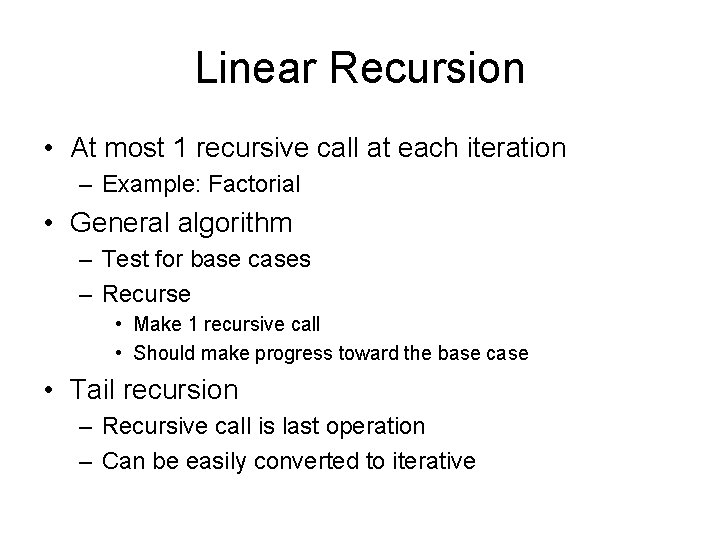Linear Recursion • At most 1 recursive call at each iteration – Example: Factorial • General algorithm – Test for base cases – Recurse • Make 1 recursive call • Should make progress toward the base case • Tail recursion – Recursive call is last operation – Can be easily converted to iterative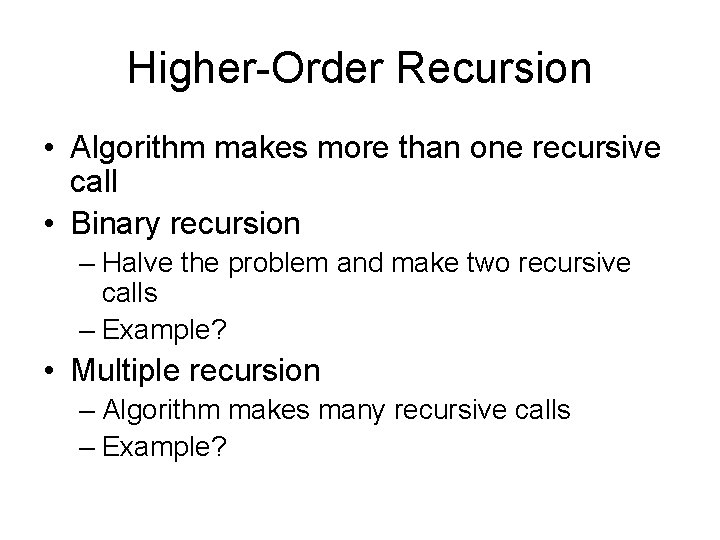Higher-Order Recursion • Algorithm makes more than one recursive call • Binary recursion – Halve the problem and make two recursive calls – Example? • Multiple recursion – Algorithm makes many recursive calls – Example?Rules of Recursion 1. Base cases. You must always have some bases cases, which can be solved without recursion. 2. Making progress. For the cases that are to be solved recursively, the recursive call must always be to a case that makes progress toward a base case. 3. Design rule. Assume that all the recursive calls work. 4. Compound interest rule. Never duplicate work by solving the same instance of a problem in separate recursive calls.Examples • Give an algorithm that uses tail recursion to convert an array of elements into their absolute value. • Describe a binary recursive method for search for an element X in an unsorted array A.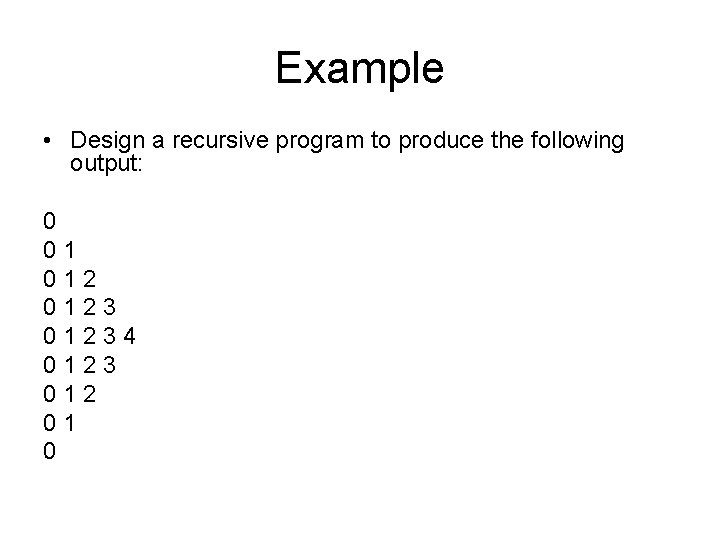Example • Design a recursive program to produce the following output: 0 01 01234 0123 012 01 0